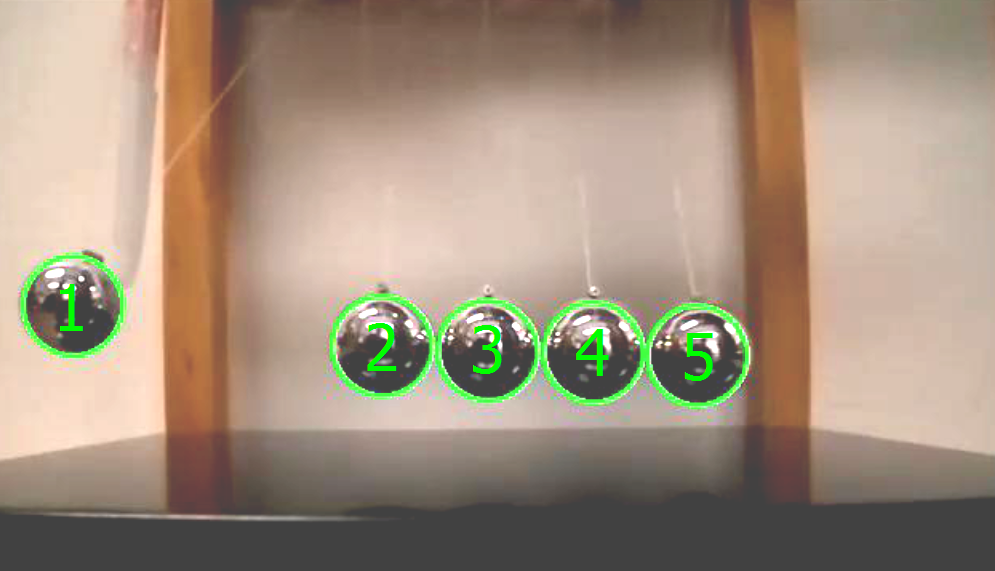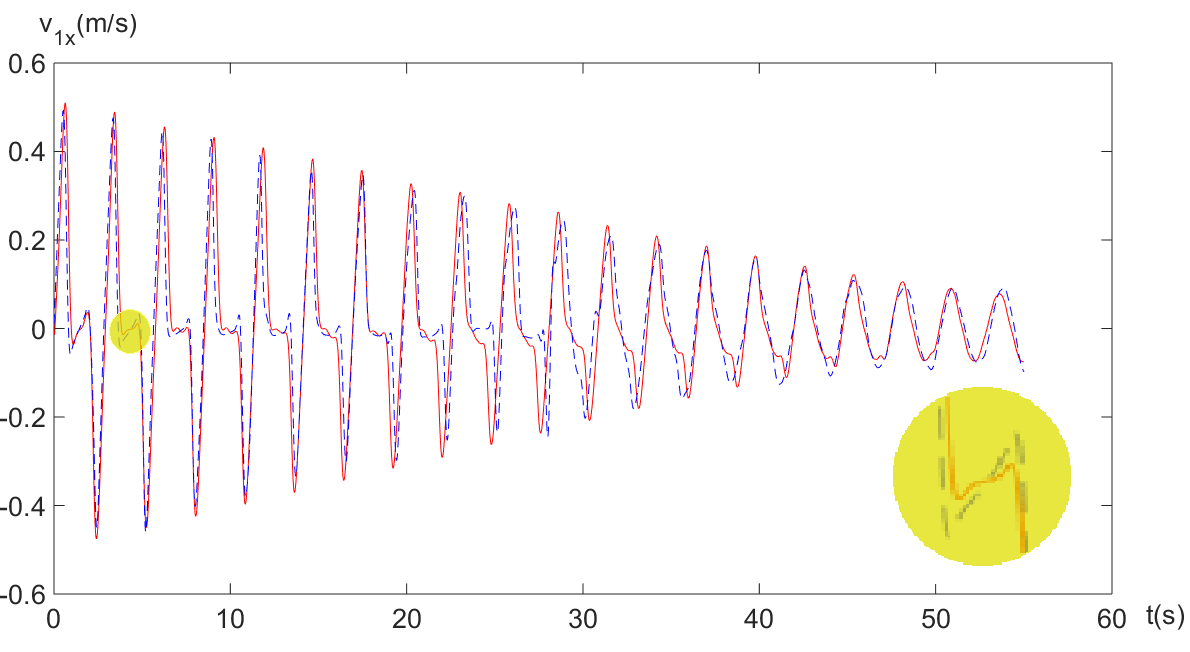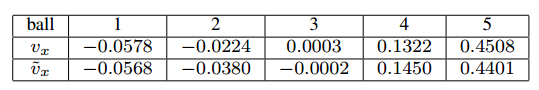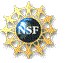# Robotics Laboratory

## Newton's Cradle with Multiple Impacts: Simulation and Experiment

Translation: Hindi

In this topic, we model a multi-body collision in the impulse space as a state transition diagram. Each state describes a different topology of the collision characterized by the set of instantaneously active contacts. Within a state, (normal) impulses are coupled differentially subject to relative stiffnesses at the active contact points and the strain energies stored there. Such coupling may cause restart of compression from restitution during a single impact. Impulses grow along a bounded curve with first-order continuity, and converge during the state transitions.

The introduced state transition diagram breaks down a multi-body collision into a state sequence, each representing a distinct subset of contacts that are instantaneously active during the collision. There could be up to 2^n states, many of which may not appear in the collision. We generate the transition diagram on the fly, starting with the initial state where all contacts are active. A transition leads to either a new state (to be generated) or to an already generated state. Transition from one state to another happens when either an impact finishes restitution or an inactive contact is reactivated because the two engaged objects are approaching each other again. Every contact is associated with an energetic coefficient of restitution that specifies the return of a fixed portion of the strain energy stored at the contact during compression. The impact at this contact is related to that at the other contact differentially as determined by their relative stiffness and the ratio between their stored strain energies. Since each impact may be affected by the other impact, it may go through multiple rounds of compression and restitution. The transition diagram stops as soon as the end state with no active contacts is reached.

The problem of Newton's cradle is well known for multiple impacts. The system has been studied as a benchmark for multiple impacts. A typical Newton’s cradle consists of a series of identically sized balls suspended in a metal frame so that they are just touching each other at rest. Each ball is attached to the frame by two wires of equal length angled away from each other. This restricts the pendulums’ movements to the same plane.

With no closed-form solution to the ordinary differential equations for the derivatives of energy with respect to impulse, and impulse with respect to primary impluse, the state evolution is simulated via via numerical integration. At each integration step, we check whether compression of either impact has just ended, and if so scale the corresponding strain energy accordingly to recognize the energy loss. And the state transits when the strain energy of some ball in the contact set decreases to zero during restitution. The initialization of integration is achieved as follows. The inital energies are approximated as the derivatives of impulse, and then based on differential relations they end up being described by a quadratic system, which is solved using the homotopy continuation method.

In the simulation, a chain involving five balls set up as above will be investigate based on the multiple impact model we introduced. All the balls in the chain are indentical. The contacts between the balls are treated as point contacts and modeled as Hertz contact, in which the force is represented as F = k * x^(1.5), where k is the stiffness of the spring and x is the change in the virtual spring’s length.

We simulate five scenarios of Newton’s cradle (as shown in the following videos): (1) one ball hit from right side; (2) two balls hit from right side; (3) three balls hit from right side; (4) four balls hit from right side; and (5) one ball hit from both sides. The initial angles of hitting balls are set to 45 degree. Energy dissipation are considered here, in which the energetic coefficient of restuition is set to 0.96. The ball motions begins to swing together (for the first four cases) or stops (for the fifth case) at 76.7s, 58.8s, 51.7s, 98.8s and 174.5s, respectively.

To experimentally validate our algorithm, a Newton's Cradle with string length l = 0.129m was placed on a horizontal table. The five identical balls of the cradle have radius r = 0.011m. The coefficient of restitution between any two balls is e = 0.95, and the stiffness between them is the same. The leftmost ball was initially raised and held static with oscillation angle 5π/36 as shown in the right figure below. Started at the release, a video was captured and Hough Circle Transform algorithm was applied to track the contours of five balls during their motion.

The table in the middle below compares the horizontal velocities of the five balls right after they were disengaged from the first collision in the experiment and the simulation. Interestingly, ball 1 was moving leftward slightly, and ball 4 was moving rightward with noticable velocity in both the experiment and simulation. This phenomenon cannot be explained by a simpler model based on conservation of momentum and energy only.

The experimental and simulation results are also compared along the time axis until no more impact happened and all the balls swung together. The figure on the right compares the time trajectories of the horizontal velocities of ball 1 observed in the experiment and predicted by simulation. The two trajectories match very well, except that during each cycle (shown in the inset) the ball velocity fluctuates slightly more in simulation than in the experiment. This is mainly due to some sticking effect between the balls in the experiment that is not modeled.We refer to the following papers for more on multiple impacts:This material is based upon work supported by the National Science Foundation under Grant IIS-1421034.
Any opinions, findings, and conclusions or recommendations expressed in this material are those of the author(s) and do not necessarily reflect the views of the National Science Foundation.

Last updated on April 3, 2015.